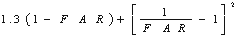Close

This page calculates the head loss coefficient and head loss of a fluid across a screen (circular metal wire mesh) inside a pipe. It is assumed that Re is greater than 103.

The head loss hL can be calculated as:

hL = kloss x (um2 / (2 x g) )

Where kloss is the head loss coefficient, um is the flow velocity in the pipe, and g is the gravitational acceleration.  kloss is calculated as:

kloss =Where FAR is the free area ratio. The free area ratio must be entered directly by the user, but is defined as:

FAR = Free Area / Total Area

References

Idel'chik, I E. and Fried, Erwin., Flow Resistance, a Design Guide for Engineers, Taylor & Francis, Washington D.C. 1989. p 265.